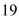Problem

# Solutions For Applied Combinatorics Chapter 1.3 Problem 3E

#### Step-by-Step Solution

Solution 1

The objective is to determine the largest possible number of vertices in the graph havingedges and degree of each vertex is at least three.

Theorem: if the number of vertices in a graph then the sum of degrees of all vertices is equal to twice the number of edges.

Now to find out the largest number of vertices in the graph having 19 edges, the degree of each vertex should be as small as possible.

Assume that the degree of each vertex is three as it is the minimum possible value.

Now, according to the theorem;

\begin{aligned} 3+3+\ldots(n \text { times }) &=2 \times 19 \\ 3 n &=38 \\ n &=12.67 \end{aligned}

But the number of vertices in the graph must be an integer and the number of vertices cannot be more than $$12.67$$

Therefore, the largest possible number of vertices in the graph must be 12 .